Level 16 Level 18
Level 17

## Ignore words

Check the boxes below to ignore/unignore words, then click save at the bottom. Ignored words will never appear in any learning session.

Ignore?
Falling factorial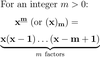Binomial coefficient (for integers)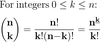Binomial coefficient (in general)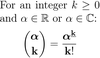Pascal's rule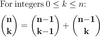Pascal's triangleBinomial formula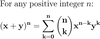Inclusion-exclusion principle (for cardinality of 2 sets)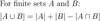Rule of sum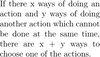Rule of product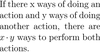Pidgeonhole principle (Dirichlet's drawer principle)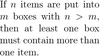Inclusion-exclusion principle (for cardinality of 3 sets)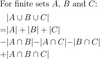Rising factorial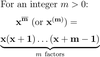Permutation (combinatorics)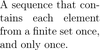n! (permutations)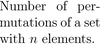k-combination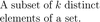n choose k (set theoretic)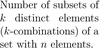Multiset (or bag)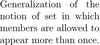n+k-1 choose k (set theoretic)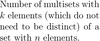Rule of sum (formula)Rule of product (formula)Inclusion-exclusion principle (for cardinality of n sets)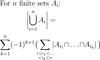Multinomial coefficient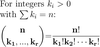Partition (of a set)